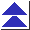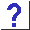# 6. Arrays and Pointers

6.1 I had the definition char a in one source file, and in another I declared extern char *a. Why didn't it work?

6.2 But I heard that char a[] was identical to char *a.

6.3 So what is meant by the ``equivalence of pointers and arrays'' in C?

6.4 If they're so different, then why are array and pointer declarations interchangeable as function formal parameters?

6.4b So arrays are passed by reference, even though the rest of C uses pass by value?

6.5 Why can't I do something like this?

```	extern char *getpass();
char str;
str = getpass("Enter password: ");
```

6.6 If you can't assign to arrays, then how can

```	int f(char str[])
{
if(str == '\0')
str = "none";
...
}
```
work?

6.6b And what about this? Isn't this an array assignment?

```	char a[] = "Hello, world!\n";
```

6.7 How can an array be an lvalue, if you can't assign to it?

6.8 Practically speaking, what is the difference between arrays and pointers?

6.9 Someone explained to me that arrays were really just constant pointers.

6.10 I'm still mystified. Is a pointer a kind of array, or is an array a kind of pointer?

6.11 I came across some ``joke'' code containing the ``expression'' 5["abcdef"] . How can this be legal C?

6.12 Since array references decay into pointers, if arr is an array, what's the difference between arr and &arr?

6.13 How do I declare a pointer to an array?

6.14 How can I set an array's size at run time?
How can I avoid fixed-sized arrays?

6.15 How can I declare local arrays of a size matching a passed-in array?

6.16 How can I dynamically allocate a multidimensional array?

6.17 Here's a neat trick: if I write

```	int realarray;
int *array = &realarray[-1];
```
I can treat array as if it were a 1-based array.

6.18 My compiler complained when I passed a two-dimensional array to a function expecting a pointer to a pointer.

6.19 How do I write functions which accept two-dimensional arrays when the width is not known at compile time?

6.20 How can I use statically- and dynamically-allocated multidimensional arrays interchangeably when passing them to functions?

6.21 Why doesn't sizeof properly report the size of an array when the array is a parameter to a function? I have a test routine

```	f(char a)
{
int i = sizeof(a);
printf("%d\n", i);
}
```
and it prints 4, not 10.

6.22 How can code in a file where an array is declared as extern (i.e. it is defined, and its size determined, in some other file) determine the size of the array? sizeof doesn't seem to work.

6.23 I want to know how many elements are in an array, but sizeof yields the size in bytes.

6.24 Is there a way to have an array of bits?